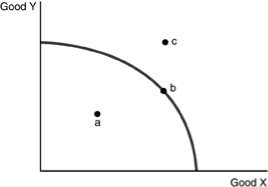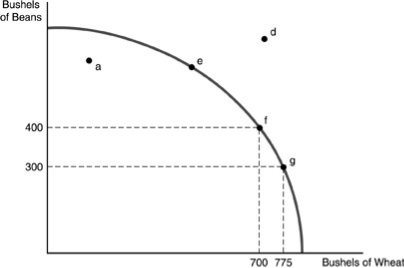• FAQ
• Contact/ Homework Answers / Economics / 51) A point inside a production possibilities curve indicates A) resources
Not my Question
Flag Content

# Question : 51) A point inside a production possibilities curve indicates A) resources

51) A point inside a production possibilities curve indicates

A) resources are not being used efficiently.

B) resources are being used very efficiently.

C) opportunity costs are constant.

D) an output combination that is unobtainable with the current resource and technology levels

52) A point on a production possibilities curve indicates

A) resources are not being used efficiently.

B) resources are being used efficiently.

C) opportunity costs are constant.

D) an output combination that can be attained only if society gets more resources or there is technological change.

53) An efficient point of production is

A) a point on the production possibilities curve.

B) a point inside the production possibilities curve.

C) the point where scarcity no longer exists.

D) the point where we are currently producing.54) Refer to the above figure. Which of the following statements is TRUE?

A) Point a is efficient, Point b is efficient, point c is inefficient.

B) Point a is unobtainable, point b is efficient and point c is inefficient.

C) Point a is inefficient, point b is efficient and point c is unobtainable.

D) All of the points are efficient.55) Refer to the above figure. Which of the following points indicates an efficient use of resources?

A) a

B) d

C) e

56) Refer to the above figure. Which of the following points indicates an inefficient use of resources?

A) a

B) d

C) e

57) Refer to the above figure. Which of the following points indicates an unobtainable point of production?

A) a

B) d

C) e

58) Refer to the above figure. The shape of the production possibility curve indicates that production of

A) wheat is characterized by increasing costs while the production of beans is characterized by decreasing costs.

B) wheat is characterized by decreasing costs while the production of beans is characterized by increasing costs.

C) both goods are characterized by increasing costs.

D) both goods are characterized by decreasing costs.

59) Refer to the above figure. Between points f and g, the opportunity cost of producing 75 more bushels of wheat is

A) 1 bushel of beans.

B) 4 bushels of beans.

C) 25 bushels of beans.

D) 100 bushels of beans.

60) Production is efficient when

A) it generates a point beyond the production possibility curve.

B) the maximum output possible is being produced given current levels of resources and technology.

C) technological change occurs.

D) the maximum amounts of the most important good are produced.

## Solution 5 (1 Ratings )

Solved
Economics 5 Months Ago 6 Views